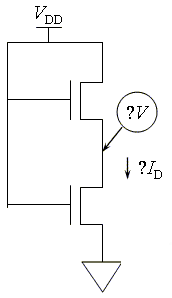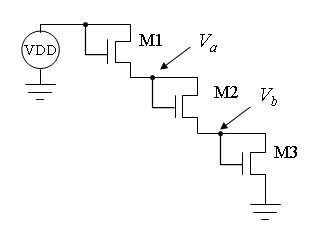# Assignment 3

Turn in your assignment on Isidore. Your report should be a Microsoft Word document or PDF. Upload all appropriate computer files associated with the assignment, such as SPICE .asc files, ELectric .jelib files and Excel spreadsheets.

Download: ON-C5 technology files and use the ON_C5.lib for all transistors.

The term NMOS transistor refers to a generic transistor of the type NMOS. Whenever you design a specific transistor in LTSpice, use the model name `CMOSN`, in agreement with the specification in the library file `ON_C5.lib`.

1. Use spice to generate a set of I-V curves for a NMOS transistor using the CMOSN model in ON_c5.lib. Make the transistor W/L = 7.2µm/0.6µm. Transfer the curves to an excel file and plot the results in excel.

2. Use spice to find the current and voltage shown for two NMOS transistors in series, shown below (VDD = 5 volts). Include the proper body connections (not shown). Make the transistors W/L = 10/2, meaning W = 3 µm and L = 0.6 µm.3. Analyze the circuit below in Spice. Make the transistors W = 8 λ and L = 2 λ where λ = 0.3 µm. Use proper body connections. VDD = 5 v. Show your SPICE circuit diagram and netlist. Find voltages Va and Vb and the current flowing through the transistors.4. Given the parameters in the table below

 VTO zero-bias threshold voltage VT0 0.8 V GAMMA body-effect parameter γ 0.6 PHI surface to bulk potential 2|φ| 0.6 V CJ0 bottom wall depletion capacitance Cj0 4 10-4 F/m2 MJ botom wall grading coefficient m 0.43 PB bottom built-in potential φ0 0.74 V

1. Find the threshold voltage if Vsb = 3V

2. Convert CJ0 to units of fF/µm2.

3. Find the bottom capacitance of the source if Vsb = 3V and AS = 50p. Note that the equivalent diode is reversed biased (VD is negative).

5. Work through tutorial 2 (see link below). Your report should include a layout figure, schematic figure, and a report of Spice analysis for the transistors.

Tutorial 2 – Layout and simulating the IV curves of PMOS and NMOS devices – electric_tutorial_2_video.wmv (33:57)

Maintained by John Loomis, last updated 29 January 2015